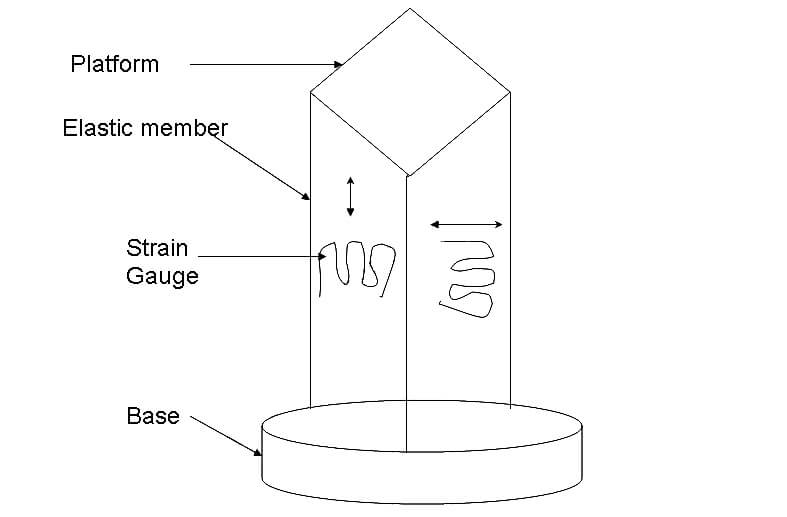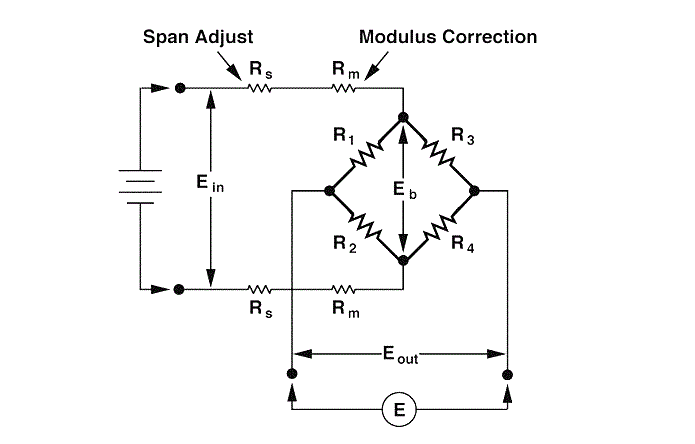A strain gauge – elastic member combination arrangement used for the measurement of load or weight is called load cell. It utilizes an elastic member as primary transducer and strain gauge as a secondary transducer.

A suitable plane area is placed on the eleastic member as a weight stand. Then, strain gauge is attached to the elastic member.The figure shown below is the Load cell Construction Diagram.On applying load, the elastic member will deform or deflect.

Since the strain gauge is attached to the elastic member, strain gauge may elongate or compress due to tension. This varies its length and in turn its resistance varies.

By measuring the variation in the resistance of the strain gauge, the applied load can be calculated.The figure shows the construction of the tensile compressive cell which is a cylinder. This arrangement uses four strain gauges, each mounted at 90 to each other.

On applying load, due to tension, two of the strain gauges experience elongation or tensile stress while the other two are subjected to compression.Since the load cell is cylinder, an axial compressive load causes a negative strain in vertical gauges and a positive strain in the circumferential gauges.

The two strains are not equal. By using bridge circuit, resistance of the strain gauge is measured and hence the unknown applied load.

The Load Cell Types are classified based on three criteria. They are

• Working principle of a load cell
• Electrical Properties of the load cell

Based on the Working Principle of the Load Cell

• Compression Principle
• Tensile based Working
• Universal
• Hollow
• Shear Based

Based on the Construction of the Load Cell

• S type Construction load cell
• Single ended shear beam
• Double ended shear beam Load cell
• Single column and Multi Column load cell
• Diaphragm/membrane

Based on the Electrical Properties of the Load cell

• Analog and Digital property based load cell
• Resistance and Capacitance Based
• Piezoelectric and Wireless Based Load cell
1.